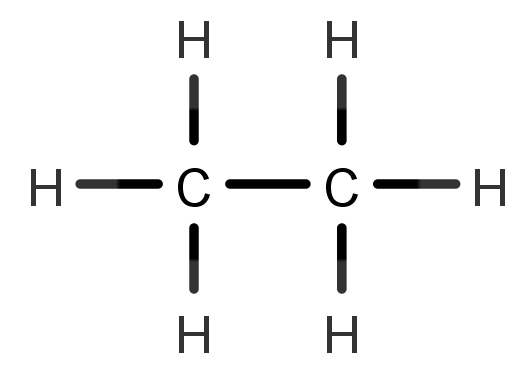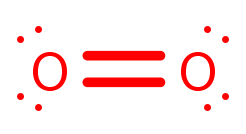# Problem: Ethane, a minority component of natural gas, burns to form carbon dioxide and water according to this reaction:2 H3C−CH3(g) + 7 O2(g) → 4 CO2(g) + 6 H2O(g)Note that the average bond energy for the breaking of a bond in CO2 is 799 kJ/mol. Use average bond energies to calculate ΔHrxn for this reaction.

###### FREE Expert Solution

To calculate the ΔH°rxn using bond energies, we’re going to use the following equation:

$\overline{){\mathbf{∆}}{\mathbf{H}}{{\mathbf{°}}}_{{\mathbf{rxn}}}{\mathbf{=}}{\mathbf{∆}}{\mathbf{H}}{{\mathbf{°}}}_{{\mathbf{reactants}}}{\mathbf{-}}{\mathbf{∆}}{\mathbf{H}}{{\mathbf{°}}}_{{\mathbf{products}}}}$

Balanced Reaction:  2 H3C−CH(g)  +  7 O(g)   →  4  CO2  (g)  +   6  H2O (g)

*always make sure that the given reaction is balanced

Draw the structure of each compound.

Reactants:

H3C−CH3• 2:97% (187 ratings)###### Problem Details

Ethane, a minority component of natural gas, burns to form carbon dioxide and water according to this reaction:

2 H3C−CH3(g) + 7 O2(g) → 4 CO2(g) + 6 H2O(g)

Note that the average bond energy for the breaking of a bond in CO2 is 799 kJ/mol. Use average bond energies to calculate ΔHrxn for this reaction.